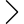155 products found
• s
• m
• l
• xl
• s
• m
• l
• xl
• s
• m
• l
• xl
• s
• m
• l
• xl
• 2xl
EXPOSED SEAMS PANEL T-SHIRT
\$179.00 \$161.00
• s
• m
• l
• xl
• s
• m
• l
• xl
• s
• m
• l
• xl
MULTI LAYERED DENIM COAT
\$369.00 \$332.00
• s
• m
• l
• xl
• s
• m
• l
• xl
• s
• m
• l
• xl
• s
• m
• l
• xl
CONTRAST PANEL LOUNGE PANTS
\$269.00 \$242.00
• s
• m
• l
• xl
• s
• m
• l
• xl
CONTRAST PANEL LOUNGE PANTS
\$269.00 \$242.00
• s
• m
• l
• xl
• s
• m
• l
• xl
CONTRAST PANEL LOUNGE PANTS
\$269.00 \$242.00
• s
• m
• l
• xl
• s
• m
• l
• xl
LAYERED PANEL CARGO PANTS
\$319.00 \$287.00
• s
• m
• l
• xl
• s
• m
• l
• xl
LAYERED PANEL CARGO PANTS
\$319.00 \$287.00
• s
• m
• l
• xl
• s
• m
• l
• xl
• s
• m
• l
• xl
• s
• m
• l
• xl
• s
• m
• l
• xl
• s
• m
• l
• xl
• s
• m
• l
• xl
• s
• m
• l
• xl
• s
• m
• l
• xl
• s
• m
• l
• xl
• 1
• 2
• 3
• 4
•